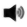# MATHS

MATHEMATICS CLUB

CLASSES VII - X

The Mathematics Department of Arwachin International School started the Mathematics club for the session 2014-15 with 12 students from classes VII –X.

The students of the club work as a team and perform many application based activities and projects on various concepts like: The square root spiral, inequalities of a triangle, area of a triangle and parallelogram, basic proportionality theorem, sum of the opposite angles of a quadrilateral, the Pythagoras theorem, tangents to a circle, solving a quadratic equation, multiplication and division of integers , rotational symmetry, identifying an AP etc. In fact, last year the students of class IX had used the concept of perfect cubes to make a cube wheel.

The students of class VII have make 3-D models using nets and other available lab materials. Also, they use the tangrams to make different shapes.

In the club, the students also prepare for the Olympiads in a competitive spirit by encouraging each other to attempt various application based problems.You Are Visitor Number 7590092
"The most wasted of all days is one without laughter. ~ E E Cummings "